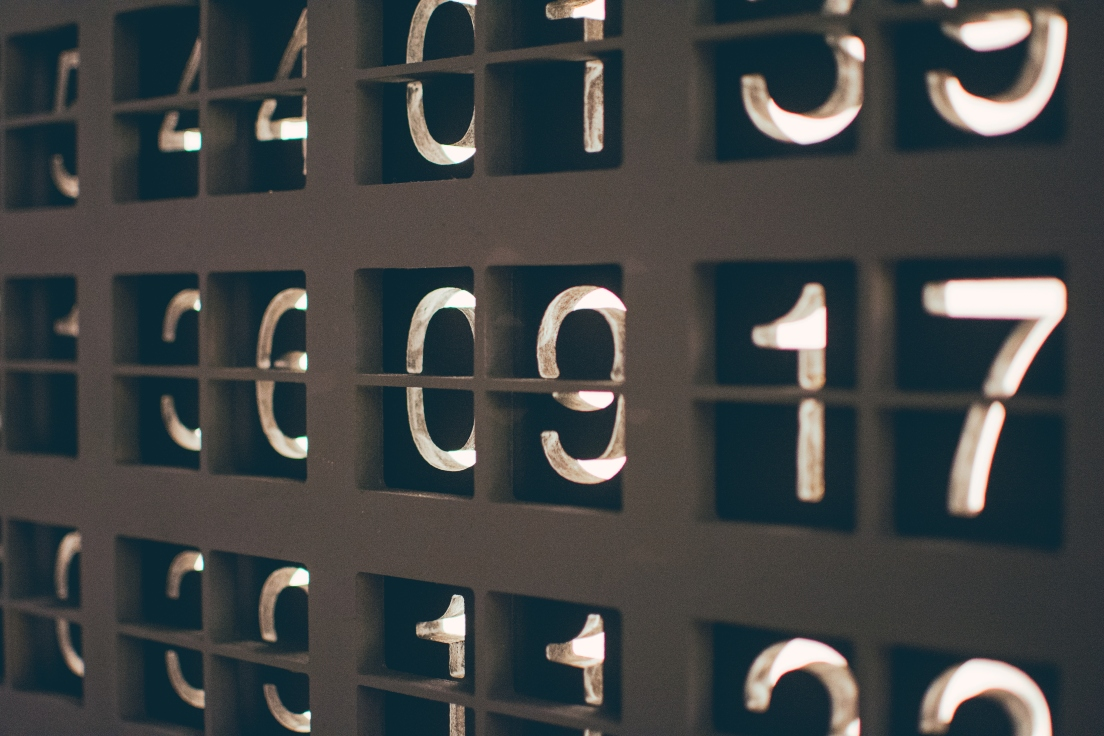# How to Format a Number to a Specific Number of Decimal Places in JavaScript

· Web Dev Zone · Tutorial
Save
22.21K ViewsIn this tutorial, we are going to learn about formatting a number to specific decimal places in JavaScript using the `toFixed()` method.

Consider that we have a number like this.

JavaScript

x

1
`const num  = 123.1390;`

Now, we need to format the above number according to specific decimal places like `123.12` or `123.139`.

## Using toFixed() Method

The `toFixed()` method formats a number and returns the string representation of a number. Be default the `toFixed()` method removes the fractional part.

It also accepts the optional argument called `digits`, which means we need to specify the number of digits after the decimal point.

Let’s see an example:

JavaScript

`xxxxxxxxxx`
1

1
`const num  = 123.1390`
2

3
`            // fractional part is removed`
4
`console.log(num.toFixed()); // "123"`

You may also like: How JavaScript Actually Works: Part 1.

Now, you can see that our number is converted to a string representation. Because of this, we need to convert the string back to a number by adding `+` operator.

JavaScript

`xxxxxxxxxx`
1

1
`console.log(+num.toFixed()); // 123`

## Formatting Number to Two Decimal places

To format a number to two decimal places we need to pass `2` as an argument to the `toFixed()` method.

JavaScript

`xxxxxxxxxx`
1

1
`const num  = 123.1390`
2

3
`console.log(+num.toFixed(2)); // 123.13`

Similarly, we can format a number according to our needs like this:

JavaScript

x
11

1
`const num  = 123.1390`
2

3
`          // 1 decimal place`
4
`console.log(+num.toFixed(1)); // 123.1`
5

6
`          // 2 decimal places`
7
`console.log(+num.toFixed(2)); // 123.13`
8

9

10
`          // 3 decimal places`
11
`console.log(+num.toFixed(3)); // 123.139`